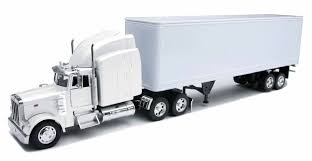Reading 10  Common Probability Distributions IntroductionIn nearly all investment decisions we work with random variables. The return on a stock and its earnings per share are familiar examples of random variables. To make probability statements about a random variable, we need to understand its probability distribution. A probability distributionspecifies the probabilities of the possible outcomes of a random variable.

In this reading, we present important facts about four probability distributions and their investment uses. These four distributions—the uniform, binomial, normal, and lognormal—are used extensively in investment analysis. They are used in such basic valuation models as the Black–Scholes–Merton option pricing model, the binomial option pricing model, and the capital asset pricing model. With the working knowledge of probability distributions provided in this reading, you will also be better prepared to study and use other quantitative methods such as hypothesis testing, regression analysis, and time-series analysis.

After discussing probability distributions, we end the reading with an introduction to Monte Carlo simulation, a computer-based tool for obtaining information on complex problems. For example, an investment analyst may want to experiment with an investment idea without actually implementing it. Or she may need to price a complex option for which no simple pricing formula exists. In these cases and many others, Monte Carlo simulation is an important resource. To conduct a Monte Carlo simulation, the analyst must identify risk factors associated with the problem and specify probability distributions for them. Hence, Monte Carlo simulation is a tool that requires an understanding of probability distributions.

Before we discuss specific probability distributions, we define basic concepts and terms. We then illustrate the operation of these concepts through the simplest distribution, the uniform distribution. That done, we address probability distributions that have more applications in investment work but also greater complexity.

If you want to change selection, open original toplevel document below and click on "Move attachment"